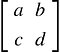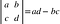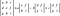# Matrix Operations in Java: Determinants

## Computing the determinant recursively

In my never-ending effort to translate all of that linear algebra I toiled over in college into functioning Java code (make fun of it on github), I came across one operation that gave me a perfect opportunity to use recursion: computing the determinant of a square matrix.

First, we’re going to talk a little bit about the determinant, so we know what computation we’re dealing with. After that, we’ll implement it recursively in Java in the class––this version is readable, but computationally expensive for large matrices. If you want to cut to the chase and see the code, feel free to check it the full class on github. It’s a work in progress!

# What is the Determinant?

Without getting too bogged down in the details (we’ve got Wikipedia for that), the determinant of a square matrix is a real number computed from the entries of the matrix. This number gives a very general idea of the nature of the transformation represented by that matrix. And when I say, “A very general idea,” I mean that you can tell whether the volume of regions in the vector space will stretch or shrink, whether orientation of the vector space will flip under the transformation (if the determinant is positive or negative), and whether or not the transformation is invertible (if the determinant is zero or non-zero). This is greatly oversimplifying the matter, and probably gave all linear algebra professors a heart attack, so for that, I send my condolences.

If you are unfamiliar with determinants and would like to develop a high-level intuition, I highly recommend the video on determinants from 3Blue1Brown’s wonderful Essence of Linear Algebra series.

# How do we compute the determinant?

The answer: It depends on the dimensions of the matrix. The determinant is only defined for square matrices, so as we will see once we get to the method, we only need to proceed if the number of rows is equal to the number of columns.

## Case 1: A 1-by-1 matrix

In the case of a 1-by-1 matrix, we have only one entry, and the determinant is equal to that entry.

## Case 2: A 2-by-2 matrix

In the case of a 2-by-2 matrix, like this one:A 2-by-2 matrix

The determinant is defined as follows:The determinant of a 2-by-2 matrix

Just a note on notation––we denote a matrix as an array of numbers surrounded by square brackets, and to denote the determinant, we replace the square brackets with vertical bars.

For our recursive solution, these will be our base cases.

## Case 3: An n-by-n matrix, where n > 2

Although there are multiple ways to compute the determinant of a larger matrix, we’re going to the Laplace expansion, also known as cofactor expansion. To simplify the implementation, we’ll always be expanding along the first row, but this expansion can work along any row or column. In practice, when working by hand, it’s a good idea to expand along a column or row with lots of zeroes in it.

Let’s motivate this explanation by looking how we’ll proceed in the case of an arbitrary 3-by-3 matrix:Determinant of a 3-by-3 matrix

There are a few things going on in this definition that we’ll need to accomplish.

1. We need to be able to compute a 2-by-2 matrix (check!)
2. We need to be able to remove the i-th row and the j-th column, in order to extract specific 2-by-2 matrices from our 3-by-3 matrix. Each of these smaller matrices are known as a minor matrix.
3. We need to iterate over the first row, multiplying the entry at by the determinant of the 2-by-2 matrix created by dropping row and column .
4. We need to add up these products with alternating signs.

Once we’ve nailed down the 3-by-3 matrix, we can compute the determinant of a 4-by-4 matrix as follows:

In other words:

1. We need to be able to compute a 3-by-3 matrix (check!)
2. Extract a specific minor matrix by dropping a specified row and column.
3. Multiply each entry in the first row by the determinant of a specific minor matrix.
4. Add up these products with alternating signs.

Hopefully, you’re catching the pattern, and can see the Case 2 is actually just a specific instance of Case 3, so the same logic applies. Here are the general steps for computing the determinant of an n-by-n matrix:

1. We need to be able to compute a (n-1)-by-(n-1) matrix (check, starting at 1-by-1 and continuing via induction!)
2. Drop a specific row and a specific column to create a minor matrix.
3. We need to iterate over the first row, multiplying the entry at by the determinant of the (n-1)-by-(n-1) matrix created by dropping row and column .
4. Add up these products with alternating signs.

Because our n-by-n determinant relies on the (n-1)-by-(n-1)th determinant, we can handle this recursively.

# Recursive Implementation in Java

To keep the focus on the recursion at work, we have a couple of things that need to be explained:

1. accepts a matrix and returns if the values returned by and are equal. All of these methods have both and instance methods ( and )––The main functionality is carried by the method, and the instance method simply contains a call to the method, passing as an argument .I tend to prefer nesting calls to methods over chaining calls to instance methods (thinking functionally, rather than OOP-ally), but this is personal preference. I use instance calls to condense my code for readability in longer expressions.
2. returns the entry in the matrix at . Again, I’ve included both and instance methods, so you’ll see both and in this code.
3. To facilitate the alternating signs, we have a factor of in our loop. Technically, this should be , but since we’re iterating over 0-th row, I have omitted the from this expression.
4. Both and return a copy of the object, with the specified row and column dropped.

So, without further ado, we have everything we need for our recursive method:

`public double determinant() {    return Matrix.determinant(this);}public static double determinant(Matrix m) {          if (!Matrix.isSquare(m)) {                 throw new IllegalArgumentException(        "Determinant undefined for non-square matrices");          }    double determinant = 0;                if (Matrix.getNumRows(m) == 1) {        determinant = Matrix.getEntry(m, 0, 0);          } else {        for (int col = 0; col < m.getNumColumns(); col++) {                     determinant += Math.pow(-1, col) *                                             m.getEntry(0, col) *                                                         m.minorMatrix(0, col).determinant();                 }          }           return determinant;   }`

The method first checks that the matrix is actually square.

If it is, it checks the dimensions to see if the base case (1-by-1) is met. If so, it returns the only entry in the .

If not, the method iterates over the first row of the , multiplying each entry by the cofactor, which is the determinant of the created by dropping the row and column that intersect at that entry. A running total is created with alternating signs, and eventually returned by the method.

# Final thoughts: Easy to implement, nightmare to use

Although the logic and implementation of this recursive approach is more or less straightforward, the performance prevents it from being the best choice in application.

To demonstrate this, we can write a quick test that times the computation of the determinant for larger and larger matrices.

`public class TestDeterminant {  public static void main() {    double[][] entries;    Matrix m;    long startTime, stopTime;           for (int n = 1; n < 15; n++) {      entries = new double[n][n];                  for (int i = 0; i < n; i++) {          for (int j = 0; j < n; j++) {            entries[i][j] = (int)(Math.random() * 100);          }        }                  m = new Matrix(entries);                  startTime = System.nanoTime();        System.out.println(n + ": Determinant =" + m.determinant());        stopTime = System.nanoTime();                  System.out.println("Time to compute: "           + (stopTime-startTime)/1000000000.0 + "\n");        }    }}`

Because I wanted to publish this post today (and not next month), I’ll just include output from the first eleven iterations:

`1: Determinant = 22.0Time to compute: 0.0017122782: Determinant = 1416.0Time to compute: 5.02671E-43: Determinant = 50983.0Time to compute: 1.07971E-44: Determinant = -6111005.0Time to compute: 5.90001E-45: Determinant = 3.7196212E8Time to compute: 0.0012946366: Determinant = 5.1611999688E10Time to compute: 0.0031718987: Determinant = -1.31321831346E12Time to compute: 0.0127575748: Determinant = -1.490499465611603E15Time to compute: 0.0790067589: Determinant = -1.8423702281090192E16Time to compute: 0.54807193310: Determinant = -1.058731125176544E18Time to compute: 4.88745859711: Determinant = 5.11833707950967E20Time to compute: 54.786274411`

For matrices smaller than 10-by-10, the determinant can be computed in less than one second, but computation becomes prohibitively slow above this, and even the Wikipedia article, which contains a Python implementation, has a special section about how unscalable this approach is––O(n!). That means a 15-by-15 would take around 3 weeks.

Fortunately, there are significantly faster implementations––especially if the is or . I look forward to exploring these optimizations in future posts.

Data Scientist, Math Enthusiast, Programmer and General Nerd. www.linkedin.com/in/danhalesprogramming

## More from Dan Hales

Data Scientist, Math Enthusiast, Programmer and General Nerd. www.linkedin.com/in/danhalesprogramming